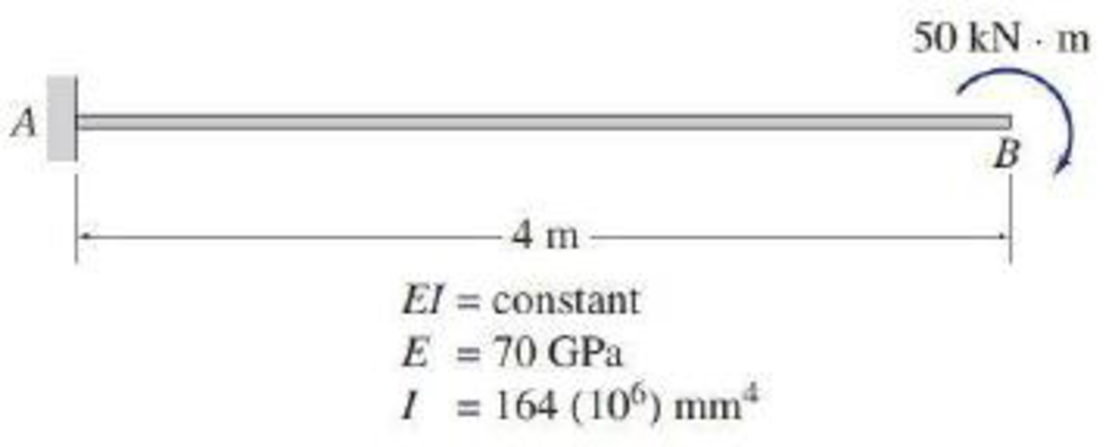# 7.22 and 7.23 Determine the deflection at point B of the beam shown in Figs. P7.20 and P7.21 by the virtual work method. Use the graphical procedure (Table 7.6) to evaluate the virtual work integrals. FIG. P7.21, P7.23, P7.58

#### Solutions

Chapter
Section
Chapter 7, Problem 23P
Textbook Problem
199 views

## 7.22 and 7.23 Determine the deflection at point B of the beam shown in Figs. P7.20 and P7.21 by the virtual work method. Use the graphical procedure (Table 7.6) to evaluate the virtual work integrals.FIG. P7.21, P7.23, P7.58

To determine

Find the deflection at point B of the beam using virtual work method.

### Explanation of Solution

Given information:

The beam is given in the Figure.

The value of E is 70 GPa and I is 164(106)mm4.

Apply the sign conventions for calculating reactions, forces, and moments using the three equations of equilibrium as shown below.

• For summation of forces along x-direction is equal to zero (Fx=0), consider the forces acting towards right side as positive (+) and the forces acting towards left side as negative ().
• For summation of forces along y-direction is equal to zero (Fy=0), consider the upward force as positive (+) and the downward force as negative ().
• For summation of moment about a point is equal to zero (Matapoint=0), consider the clockwise moment as negative and the counter clockwise moment as positive.

Calculation:

Consider the real system.

Sketch the real system of the beam as shown in Figure 1.

Refer to Figure 1.

Find moment at A.

Summation of moments about A is equal to 0.

MA=0MA50=0MA=50kNm

Sketch the moment diagram of the real system M as shown in Figure 2.

Consider the virtual system.

Remove all the real loads and apply unit load where the point to find the deflection.

Let the bending moment due to virtual load be Mv.

Sketch the virtual system of the beam with unit load at point B as shown in Figure 3.

Refer to Figure 1.

Find the reaction and moment at A.

Summation of forces along y-direction is equal to 0.

+Fy=0Ay1=0Ay=1

Summation of moments about A is equal to 0.

MA=0MA1(4)=0MA=4kNm

Sketch the moment diagram of the virtual system Mv as shown in Figure 4.

Find the deflection at B using the virtual work expression:

1(ΔB)=0LMvMEIdx (1)

Refer Table 7.6, “Integrals 0LMvMdx for moment diagrams of simple geometric shapes” in the textbook.

For the moment diagram in Figure 2 and Figure 4, the value of 0LMvMdx is 12Mv1M1L

### Still sussing out bartleby?

Check out a sample textbook solution.

See a sample solution

#### The Solution to Your Study Problems

Bartleby provides explanations to thousands of textbook problems written by our experts, many with advanced degrees!

Get Started

Find more solutions based on key concepts
Give examples of practices that you need to follow to be successful in college.

Engineering Fundamentals: An Introduction to Engineering (MindTap Course List)

What is a code of ethics? Give an example.

Fundamentals of Information Systems

What is RFID technology? Identify three practical uses for this technology.

Principles of Information Systems (MindTap Course List)

How can you protect against shoulder surfing?

Principles of Information Security (MindTap Course List)

What is XML, and why is it important?

Database Systems: Design, Implementation, & Management

Cloud Services Public, Private, Hybrid, and Personal Clouds (IaaS) When deciding how to host data and apps on t...

Enhanced Discovering Computers 2017 (Shelly Cashman Series) (MindTap Course List)

If your motherboard supports ECC DDR3 memory, can you substitute non-ECC DDR3 memory?

A+ Guide to Hardware (Standalone Book) (MindTap Course List)